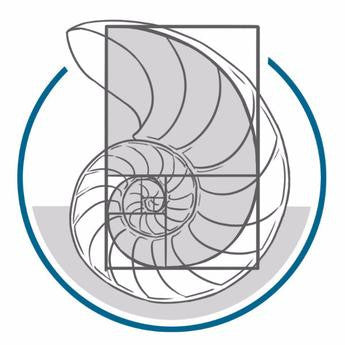# Algebra II

Regular price \$135.00

• \$675 (à la carte  tuition per class)
• - \$135 (20% non-refundable tuition deposit required to secure a seat in class)
• = \$540 balance of tuition (invoiced as monthly payments)

Course Description: This course will use the 3rd edition of the Saxon Algebra 2 textbook, providing comprehensive teaching of the fundamental aspects of problem-solving. It offers a substantial review of all topics in Algebra 1 and then moves on to cover these topics at an advanced level. Major topics include the solving and graphing of linear and quadratic equations, factoring, a variety of types of word problems, solving quadratic equations by completing the square, solving simultaneous equations with fractions and decimals, complex roots of quadratic equations, solving systems of nonlinear equations, graphing and solving a system of inequalities, exponential equations, and review of key geometry, probability and statistics topics.

Credits: 1 (Math)

Prerequisite: Students must have completed Saxon Algebra I or another algebra course

Mtg. Days: Mon., Tues., Wed., & Thur. (4 days each week)

Mtg. Times (Pacific Standard Time):

Required Materials:

Recommended: